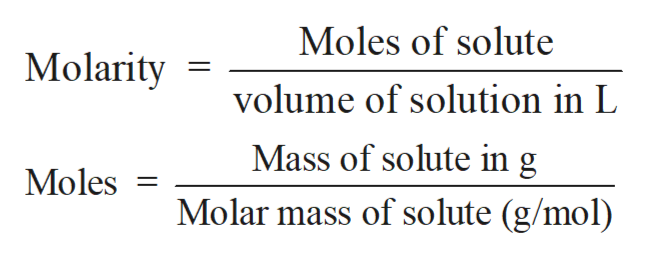1. 18.0 g of zinc is added to 100. mL of 0.200M HBr.a) Will all of the zinc dissolve?b) What will be the concentration of Br- in the resulting solution(after any reaction takes place)?

Question

1. 18.0 g of zinc is added to 100. mL of 0.200M HBr.
a) Will all of the zinc dissolve?
b) What will be the concentration of Br- in the resulting solution(after any reaction takes place)?

Step 1

Solution stoichiometry involves the calculation of concentration of solutions in the given conditions of volumes, moles etc.

Step 2

Solution stoichiometry is mainly based on the calculation of moles and volumes. These two values are used to calculate the molarity of solution. The relation between moles, volume and molarity is as given below;help_outlineImage TranscriptioncloseMoles of solute Molarity volume of solution in L Mass of solute in g Moles Molar mass of solute (g/mol) fullscreen
Step 3

The balance reaction between Z...

Want to see the full answer?

See Solution

Want to see this answer and more?

Our solutions are written by experts, many with advanced degrees, and available 24/7

See Solution
Tagged in

Solutions